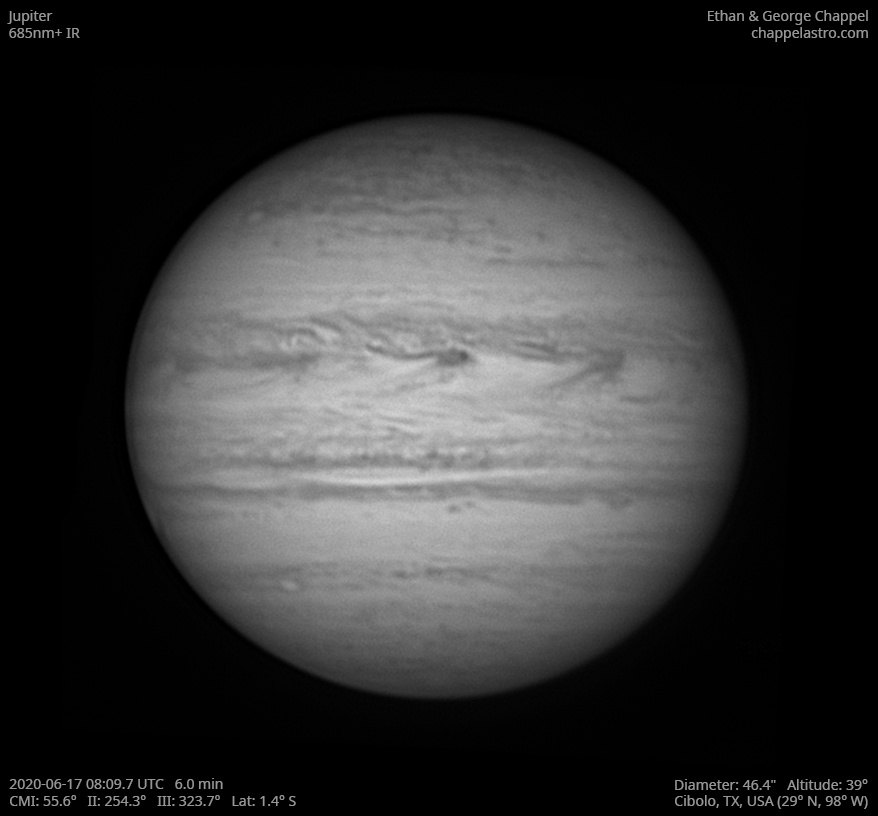# Jupiter 2020-06-17 08:09 UTC

CM1: 55.60°

CM2: 254.30°

CM3: 323.70°

CLat: -1.40°

Description

A second morning of fairly good seeing. It turns out the root of my motor focus problems was the serial cable connecting it to the controller. A new one was supposed to arrive a couple days ago, but the envelope was empty because it was improperly sealed!

Notes:

• The NEB is thinner on this hemisphere.
• White Spot Z is setting.
• The Great Red Spot is rising.
• There are two small storms on the central meridian on the boundary between the SEB and STrZ.

Equipment

ZWO ASI290MM

Celestron EdgeHD 14

Logs
```FireCapture v2.6  Settings
------------------------------------
Observer=Ethan Chappel
Location=Cibolo
Scope=Celestron C14 EdgeHD
Camera=ZWO ASI290MM
Filter=IR685
Profile=Jupiter
Diameter=46.29"
Magnitude=-2.66
CMI=56.7° CMII=255.3° CMIII=324.7°  (during mid of capture)
FocalLength=8000mm (F/22)
Resolution=0.07"
Filename=2020-06-17-0811_7-EC-IR685-Jup.ser
Date=2020_06_17
Start=08_10_47.800
Mid=08_11_47.802
End=08_12_47.805
Start(UT)=08_10_47.800
Mid(UT)=08_11_47.802
End(UT)=08_12_47.805
Duration=120.005s
Date_format=yyyy_MM_dd
Time_format=HH_mm_ss
LT=UT -6h
Frames captured=7946
File type=SER
Binning=no
ROI=724x686
ROI(Offset)=128x0
FPS (avg.)=66
Shutter=15.00ms
Gain=360 (60%)
SoftwareGain=10 (off)
AutoHisto=75 (off)
Brightness=1 (off)
Gamma=50 (off)
USBTraffic=50 (off)
AutoGain=off
FPS=100 (off)
AutoExposure=off
HighSpeed=on
Histogramm(min)=0
Histogramm(max)=218
Histogramm=85%
Noise(avg.deviation)=4.32
Limit=120 Seconds
Sensor temperature=29.7°C
FireCapture v2.6  Settings
------------------------------------
Observer=Ethan Chappel
Location=Cibolo
Scope=Celestron C14 EdgeHD
Camera=ZWO ASI290MM
Filter=IR685
Profile=Jupiter
Diameter=46.29"
Magnitude=-2.66
CMI=55.5° CMII=254.1° CMIII=323.5°  (during mid of capture)
FocalLength=8000mm (F/22)
Resolution=0.07"
Filename=2020-06-17-0809_7-EC-IR685-Jup.ser
Date=2020_06_17
Start=08_08_47.424
Mid=08_09_47.428
End=08_10_47.432
Start(UT)=08_08_47.424
Mid(UT)=08_09_47.428
End(UT)=08_10_47.432
Duration=120.008s
Date_format=yyyy_MM_dd
Time_format=HH_mm_ss
LT=UT -6h
Frames captured=7949
File type=SER
Binning=no
ROI=724x686
ROI(Offset)=128x0
FPS (avg.)=66
Shutter=15.00ms
Gain=360 (60%)
SoftwareGain=10 (off)
AutoHisto=75 (off)
Brightness=1 (off)
Gamma=50 (off)
USBTraffic=50 (off)
AutoGain=off
FPS=100 (off)
AutoExposure=off
HighSpeed=on
Histogramm(min)=0
Histogramm(max)=225
Histogramm=88%
Noise(avg.deviation)=4.43
Limit=120 Seconds
Sensor temperature=29.7°C
FireCapture v2.6  Settings
------------------------------------
Observer=Ethan Chappel
Location=Cibolo
Scope=Celestron C14 EdgeHD
Camera=ZWO ASI290MM
Filter=IR685
Profile=Jupiter
Diameter=46.29"
Magnitude=-2.66
CMI=54.2° CMII=252.9° CMIII=322.3°  (during mid of capture)
FocalLength=7950mm (F/22)
Resolution=0.08"
Filename=2020-06-17-0807_7-EC-IR685-Jup.ser
Date=2020_06_17
Start=08_06_47.031
Mid=08_07_47.040
End=08_08_47.049
Start(UT)=08_06_47.031
Mid(UT)=08_07_47.040
End(UT)=08_08_47.049
Duration=120.018s
Date_format=yyyy_MM_dd
Time_format=HH_mm_ss
LT=UT -6h
Frames captured=7999
File type=SER
Binning=no
ROI=724x686
ROI(Offset)=128x0
FPS (avg.)=66
Shutter=15.00ms
Gain=360 (60%)
SoftwareGain=10 (off)
AutoHisto=75 (off)
Brightness=1 (off)
Gamma=50 (off)
USBTraffic=50 (off)
AutoGain=off
FPS=100 (off)
AutoExposure=off
HighSpeed=on
Histogramm(min)=0
Histogramm(max)=233
Histogramm=91%
Noise(avg.deviation)=4.47
Limit=120 Seconds
Sensor temperature=29.8°C
```